# Chapter 2 Mathematical Modeling of Chemical Processes Mathematical

• Slides: 39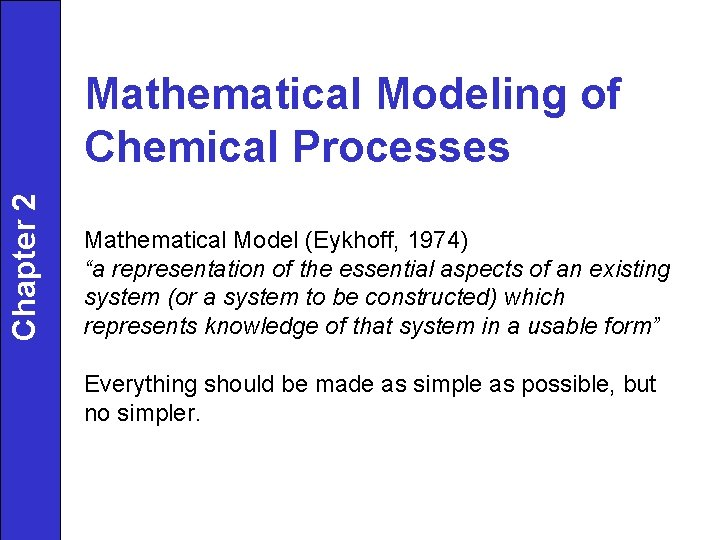Chapter 2 Mathematical Modeling of Chemical Processes Mathematical Model (Eykhoff, 1974) “a representation of the essential aspects of an existing system (or a system to be constructed) which represents knowledge of that system in a usable form” Everything should be made as simple as possible, but no simpler.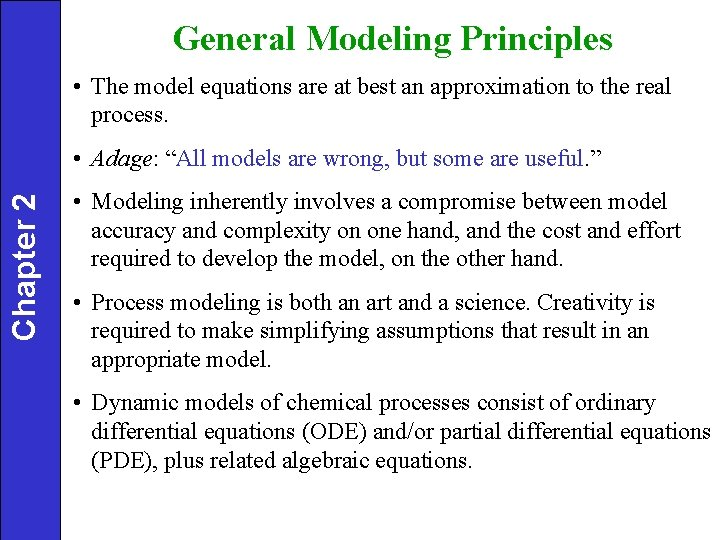General Modeling Principles • The model equations are at best an approximation to the real process. Chapter 2 • Adage: “All models are wrong, but some are useful. ” • Modeling inherently involves a compromise between model accuracy and complexity on one hand, and the cost and effort required to develop the model, on the other hand. • Process modeling is both an art and a science. Creativity is required to make simplifying assumptions that result in an appropriate model. • Dynamic models of chemical processes consist of ordinary differential equations (ODE) and/or partial differential equations (PDE), plus related algebraic equations.Chapter 2 Table 2. 1. A Systematic Approach for Developing Dynamic Models 1. State the modeling objectives and the end use of the model. They determine the required levels of model detail and model accuracy. 2. Draw a schematic diagram of the process and label all process variables. 3. List all of the assumptions that are involved in developing the model. Try for parsimony; the model should be no more complicated than necessary to meet the modeling objectives. 4. Determine whether spatial variations of process variables are important. If so, a partial differential equation model will be required. 5. Write appropriate conservation equations (mass, component, energy, and so forth).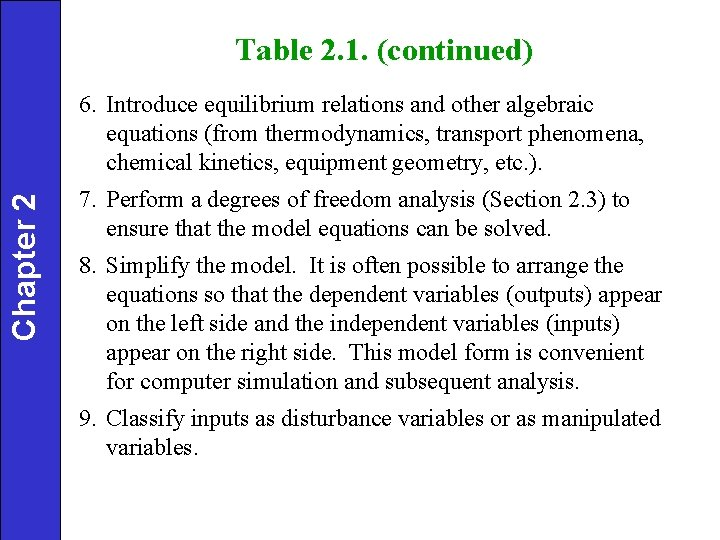Table 2. 1. (continued) Chapter 2 6. Introduce equilibrium relations and other algebraic equations (from thermodynamics, transport phenomena, chemical kinetics, equipment geometry, etc. ). 7. Perform a degrees of freedom analysis (Section 2. 3) to ensure that the model equations can be solved. 8. Simplify the model. It is often possible to arrange the equations so that the dependent variables (outputs) appear on the left side and the independent variables (inputs) appear on the right side. This model form is convenient for computer simulation and subsequent analysis. 9. Classify inputs as disturbance variables or as manipulated variables.Chapter 2 Modeling Approaches Ø Physical/chemical (fundamental, global) • Model structure by theoretical analysis § Material/energy balances § Heat, mass, and momentum transfer § Thermodynamics, chemical kinetics § Physical property relationships • Model complexity must be determined (assumptions) • Can be computationally expensive (not realtime) • May be expensive/time-consuming to obtain • Good for extrapolation, scale-up • Does not require experimental data to obtain (data required for validation and fitting)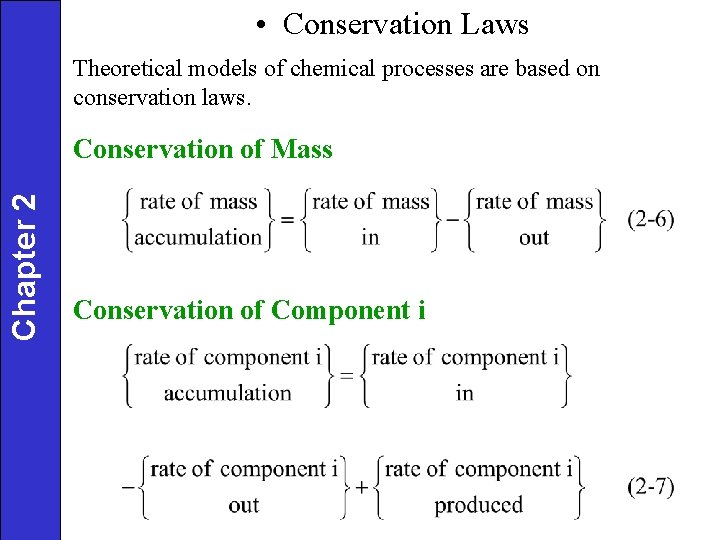• Conservation Laws Theoretical models of chemical processes are based on conservation laws. Chapter 2 Conservation of Mass Conservation of Component iConservation of Energy Chapter 2 The general law of energy conservation is also called the First Law of Thermodynamics. It can be expressed as: The total energy of a thermodynamic system, Utot, is the sum of its internal energy, kinetic energy, and potential energy: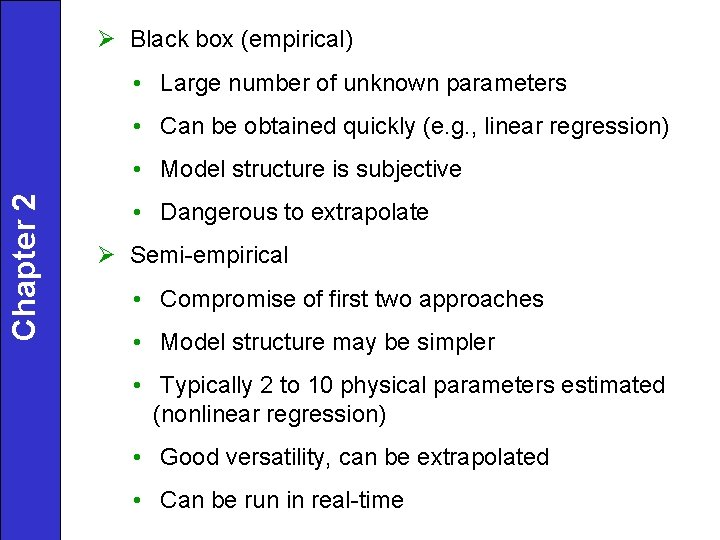Ø Black box (empirical) • Large number of unknown parameters • Can be obtained quickly (e. g. , linear regression) Chapter 2 • Model structure is subjective • Dangerous to extrapolate Ø Semi-empirical • Compromise of first two approaches • Model structure may be simpler • Typically 2 to 10 physical parameters estimated (nonlinear regression) • Good versatility, can be extrapolated • Can be run in real-time• linear regression Chapter 2 • nonlinear regression • number of parameters affects accuracy of model, but confidence limits on the parameters fitted must be evaluated • objective function for data fitting – minimize sum of squares of errors between data points and model predictions (use optimization code to fit parameters) • nonlinear models such as neural nets are becoming popular (automatic modeling)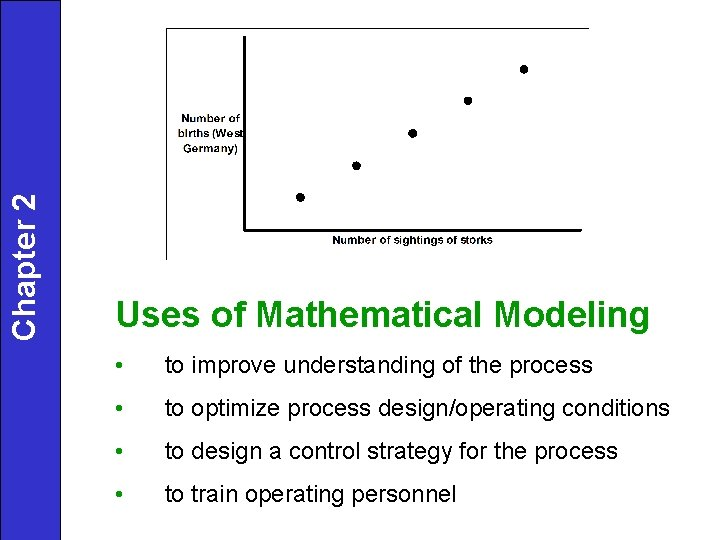Chapter 2 Uses of Mathematical Modeling • to improve understanding of the process • to optimize process design/operating conditions • to design a control strategy for the process • to train operating personnel• Development of Dynamic Models Chapter 2 • Illustrative Example: A Blending Process An unsteady-state mass balance for the blending system: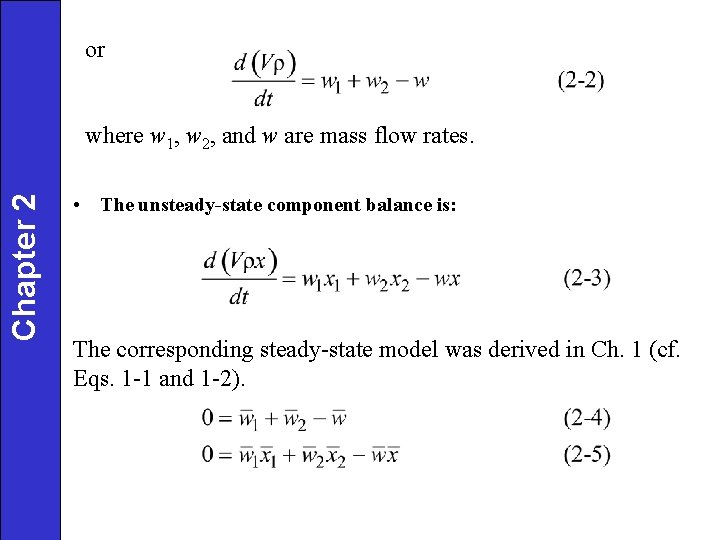or Chapter 2 where w 1, w 2, and w are mass flow rates. • The unsteady-state component balance is: The corresponding steady-state model was derived in Ch. 1 (cf. Eqs. 1 -1 and 1 -2).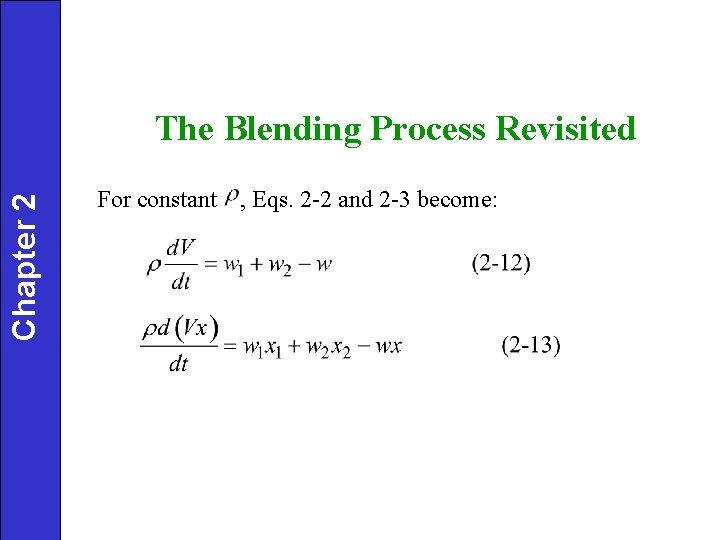Chapter 2 The Blending Process Revisited For constant , Eqs. 2 -2 and 2 -3 become: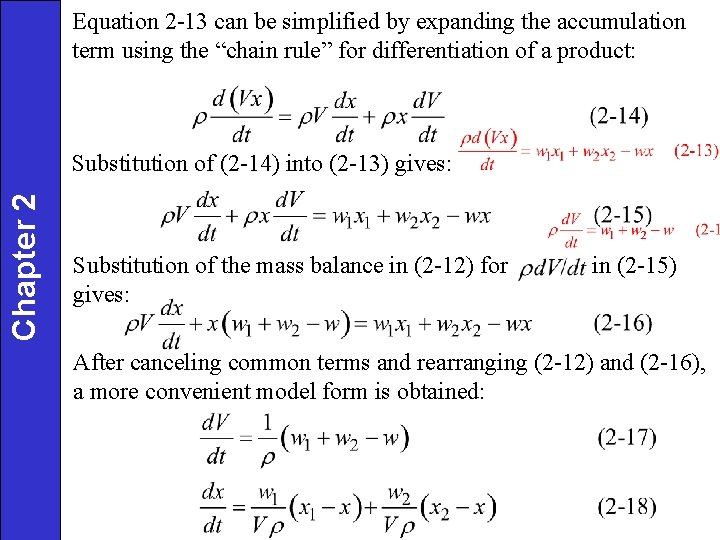Equation 2 -13 can be simplified by expanding the accumulation term using the “chain rule” for differentiation of a product: Chapter 2 Substitution of (2 -14) into (2 -13) gives: Substitution of the mass balance in (2 -12) for gives: in (2 -15) After canceling common terms and rearranging (2 -12) and (2 -16), a more convenient model form is obtained:Chapter 2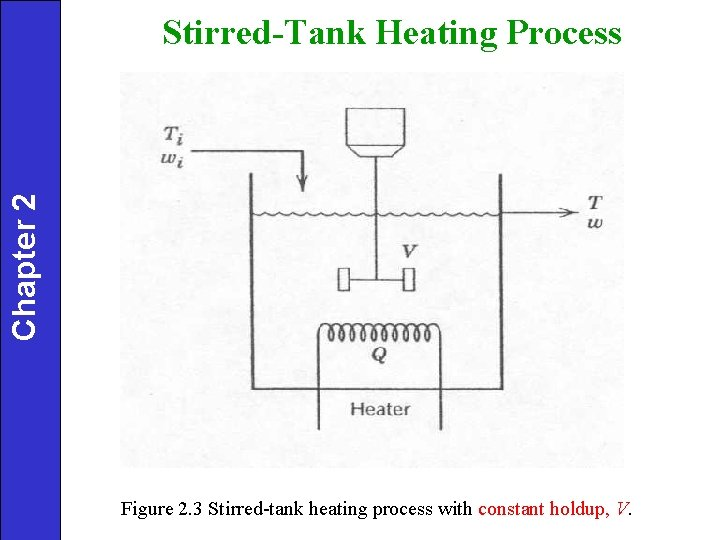Chapter 2 Stirred-Tank Heating Process Figure 2. 3 Stirred-tank heating process with constant holdup, V.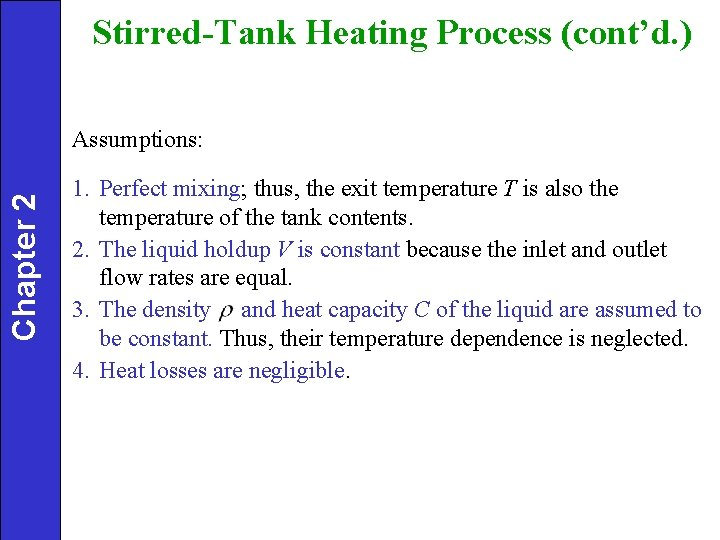Stirred-Tank Heating Process (cont’d. ) Chapter 2 Assumptions: 1. Perfect mixing; thus, the exit temperature T is also the temperature of the tank contents. 2. The liquid holdup V is constant because the inlet and outlet flow rates are equal. 3. The density and heat capacity C of the liquid are assumed to be constant. Thus, their temperature dependence is neglected. 4. Heat losses are negligible.Chapter 2 For the processes and examples considered in this book, it is appropriate to make two assumptions: 1. Changes in potential energy and kinetic energy can be neglected because they are small in comparison with changes in internal energy. 2. The net rate of work can be neglected because it is small compared to the rates of heat transfer and convection.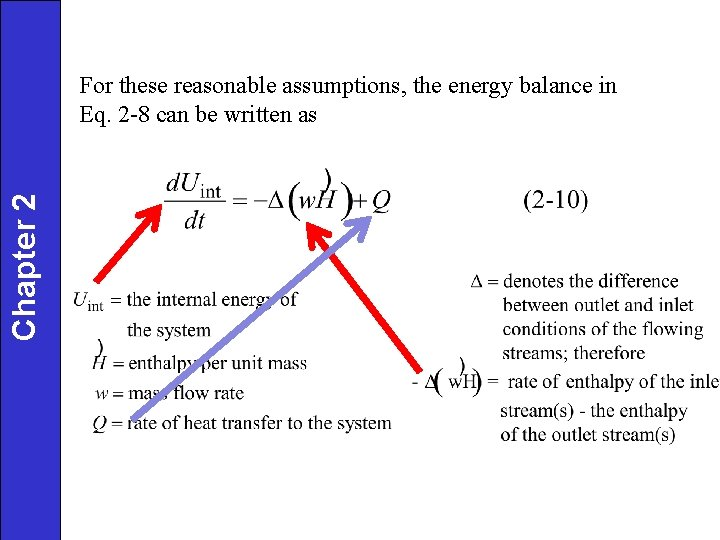Chapter 2 For these reasonable assumptions, the energy balance in Eq. 2 -8 can be written as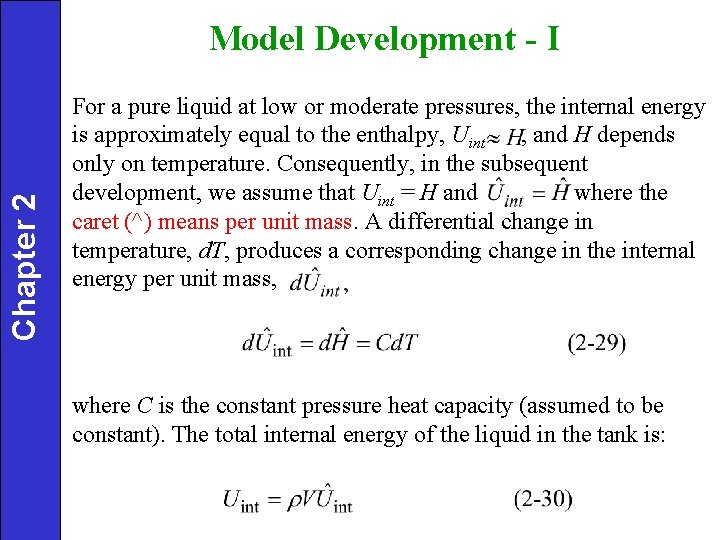Chapter 2 Model Development - I For a pure liquid at low or moderate pressures, the internal energy is approximately equal to the enthalpy, Uint , and H depends only on temperature. Consequently, in the subsequent development, we assume that Uint = H and where the caret (^) means per unit mass. A differential change in temperature, d. T, produces a corresponding change in the internal energy per unit mass, where C is the constant pressure heat capacity (assumed to be constant). The total internal energy of the liquid in the tank is: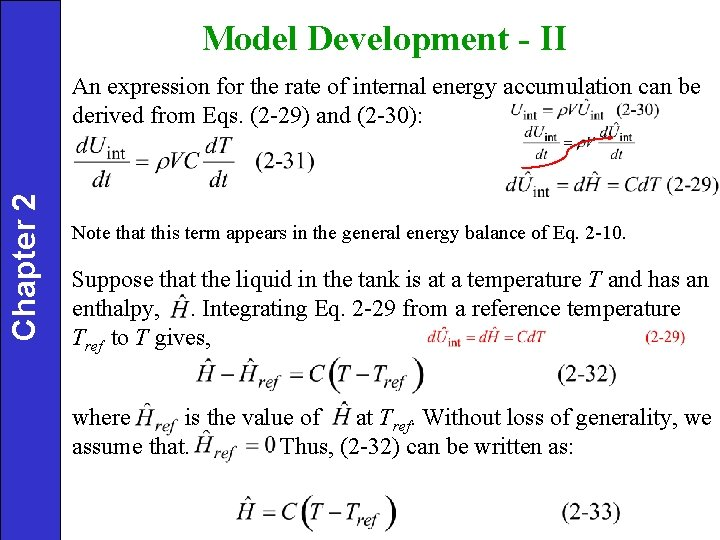Model Development - II Chapter 2 An expression for the rate of internal energy accumulation can be derived from Eqs. (2 -29) and (2 -30): Note that this term appears in the general energy balance of Eq. 2 -10. Suppose that the liquid in the tank is at a temperature T and has an enthalpy, . Integrating Eq. 2 -29 from a reference temperature Tref to T gives, where is the value of at Tref. Without loss of generality, we assume that. Thus, (2 -32) can be written as: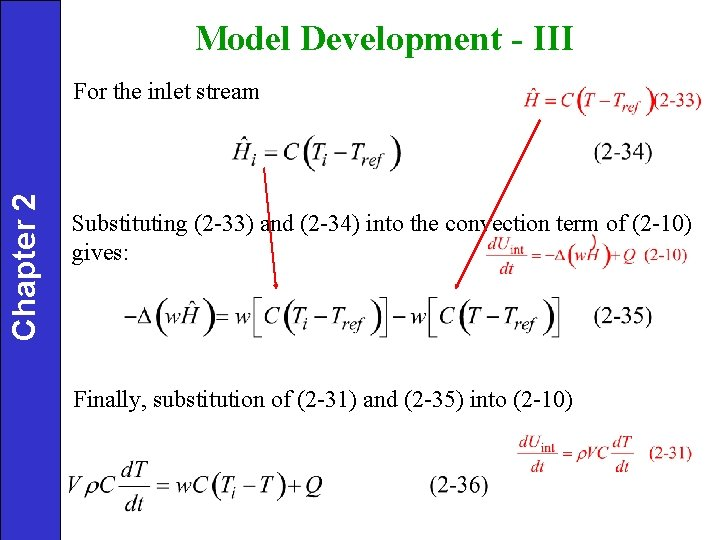Model Development - III Chapter 2 For the inlet stream Substituting (2 -33) and (2 -34) into the convection term of (2 -10) gives: Finally, substitution of (2 -31) and (2 -35) into (2 -10)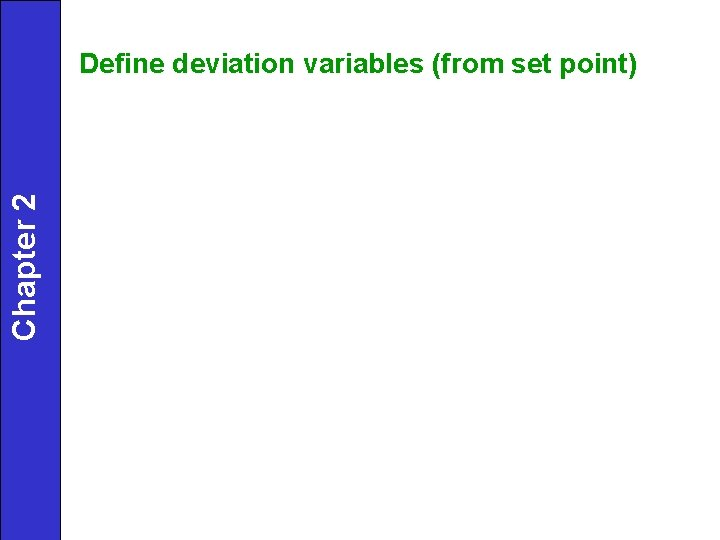Chapter 2 Define deviation variables (from set point)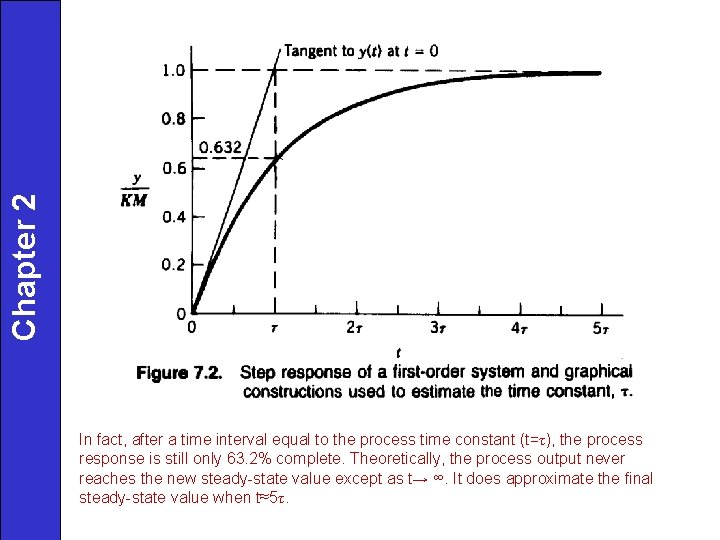Chapter 2 In fact, after a time interval equal to the process time constant (t=τ), the process response is still only 63. 2% complete. Theoretically, the process output never reaches the new steady-state value except as t→ ∞. It does approximate the final steady-state value when t≈5τ.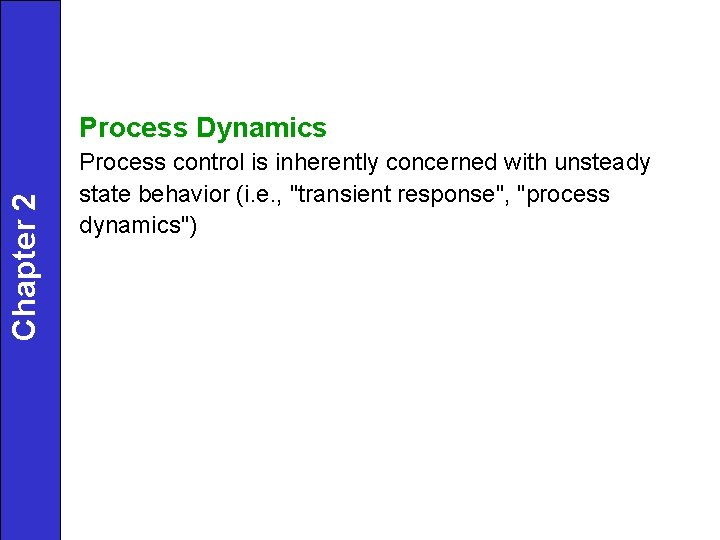Chapter 2 Process Dynamics Process control is inherently concerned with unsteady state behavior (i. e. , "transient response", "process dynamics")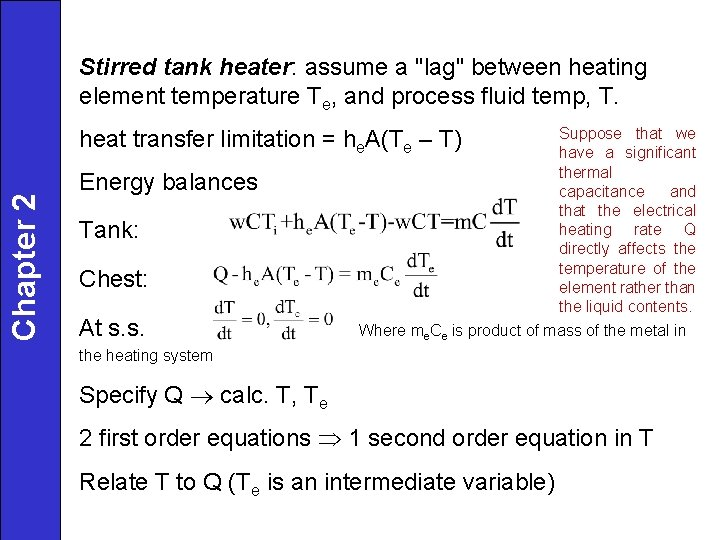Stirred tank heater: assume a "lag" between heating element temperature Te, and process fluid temp, T. Suppose that we have a significant thermal Energy balances capacitance and that the electrical heating rate Q Tank: directly affects the temperature of the Chest: element rather than the liquid contents. At s. s. Where me. Ce is product of mass of the metal in Chapter 2 heat transfer limitation = he. A(Te – T) the heating system Specify Q calc. T, Te 2 first order equations 1 second order equation in T Relate T to Q (Te is an intermediate variable)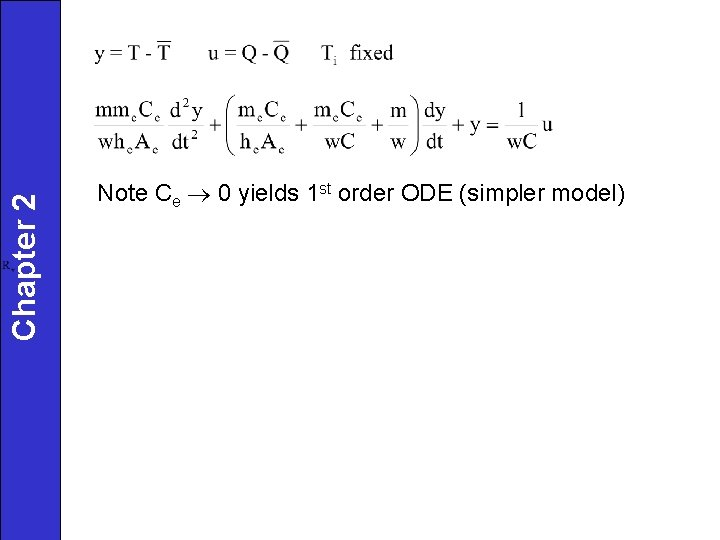Chapter 2 Note Ce 0 yields 1 st order ODE (simpler model)Rv: line resistance *Solve by substituting 2. 56 into 2. 54Chapter 2 ΔP=P-P a If nonlinear ODE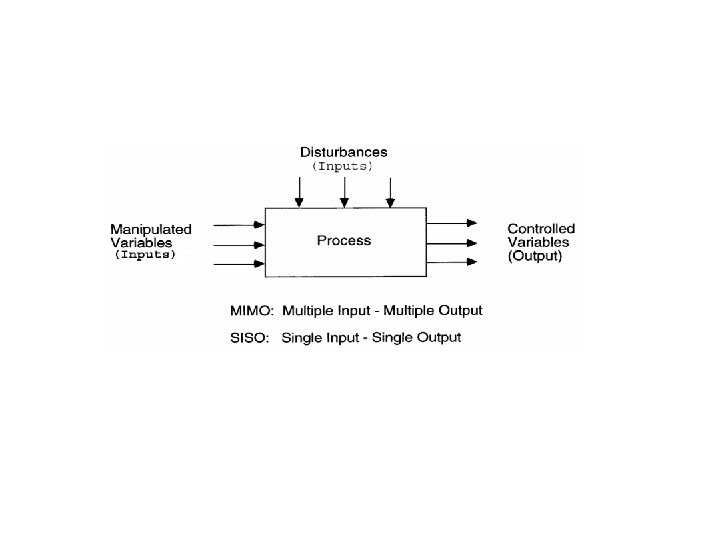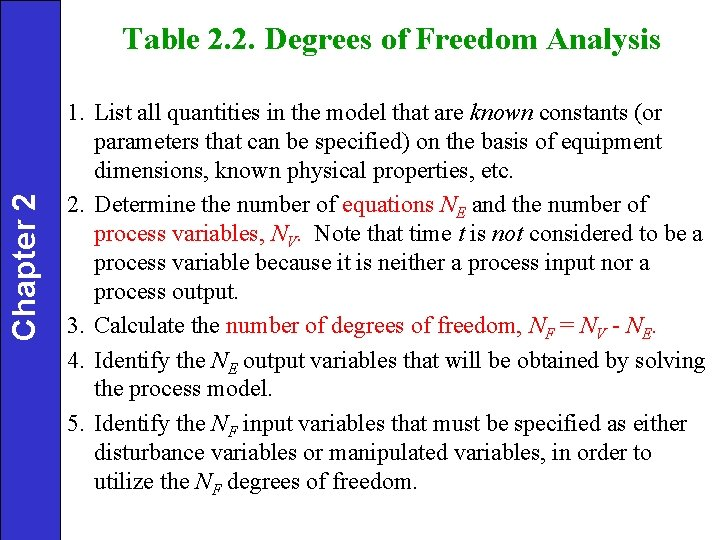Chapter 2 Table 2. 2. Degrees of Freedom Analysis 1. List all quantities in the model that are known constants (or parameters that can be specified) on the basis of equipment dimensions, known physical properties, etc. 2. Determine the number of equations NE and the number of process variables, NV. Note that time t is not considered to be a process variable because it is neither a process input nor a process output. 3. Calculate the number of degrees of freedom, NF = NV - NE. 4. Identify the NE output variables that will be obtained by solving the process model. 5. Identify the NF input variables that must be specified as either disturbance variables or manipulated variables, in order to utilize the NF degrees of freedom.Degrees of Freedom Analysis for the Stirred-Tank Model: 3 parameters: Chapter 2 4 variables: 1 equation: Eq. 2 -36 Thus the degrees of freedom are NF = 4 – 1 = 3. The process variables are classified as: 1 output variable: T 3 input variables: Ti, w, Q For temperature control purposes, it is reasonable to classify the three inputs as: 2 disturbance variables: Ti , w 1 manipulated variable: QExample 2. 2 • Analyze the degree of freedom for the blending model of for special condition where volume V constant.Solution • 2 parameters: V, ρ • 4 variables (Nv=4): x, x 1, w 2 • 1 equation(NE=1) • Degree of freedom NF=4 -1=3 So we must identify three input variables that can be specified as function of time in order for the equation to have a unique soluation.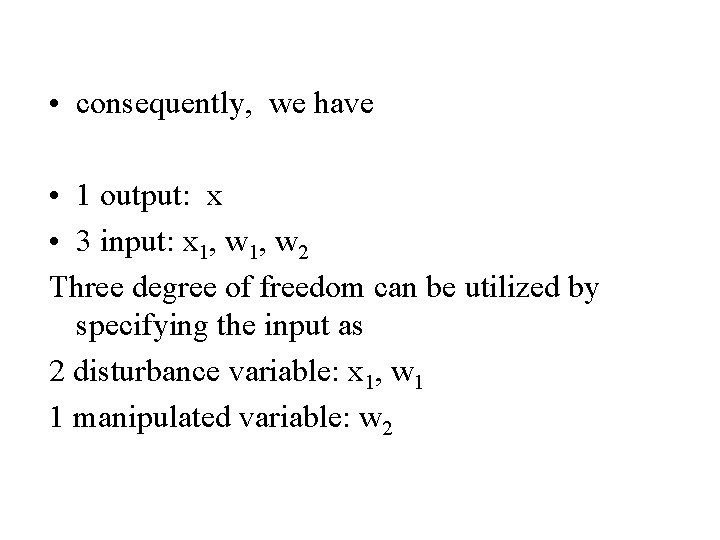• consequently, we have • 1 output: x • 3 input: x 1, w 2 Three degree of freedom can be utilized by specifying the input as 2 disturbance variable: x 1, w 1 1 manipulated variable: w 2Example 2. 3 • Analyze the degree of freedom of the blending system model in Is this set of equation linear or nonlinear, according to the usual working definitionSolution • Volume is variable rather than a constant parameter • 1 parameter: ρ • 7 variables (Nv=7): V, x, x 1, x 2, w, w 1, w 2 • 2 equations: NE=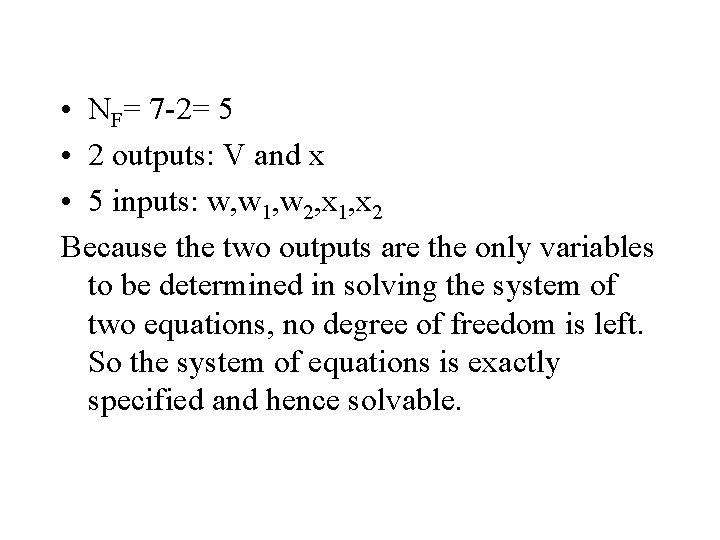• NF= 7 -2= 5 • 2 outputs: V and x • 5 inputs: w, w 1, w 2, x 1, x 2 Because the two outputs are the only variables to be determined in solving the system of two equations, no degree of freedom is left. So the system of equations is exactly specified and hence solvable.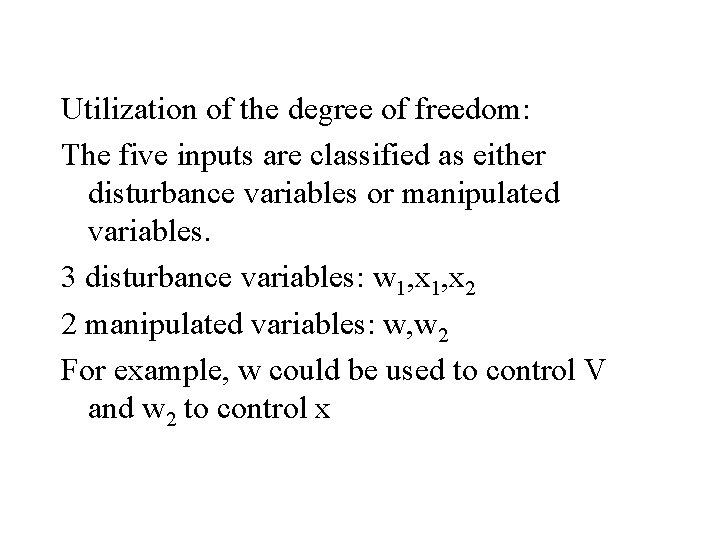Utilization of the degree of freedom: The five inputs are classified as either disturbance variables or manipulated variables. 3 disturbance variables: w 1, x 2 2 manipulated variables: w, w 2 For example, w could be used to control V and w 2 to control x